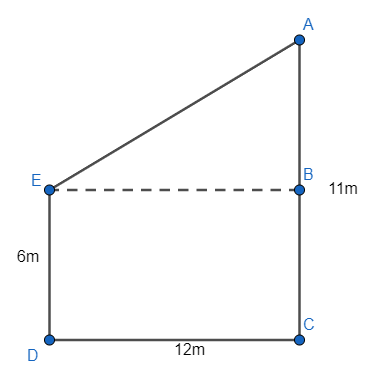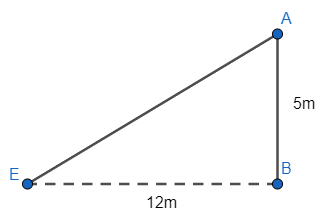QUESTION

# Two poles of height 6m and 11m stands on a plane ground, if the distance between their feet is 12m. Find the distance between their tops.

Hint: Draw a neat diagram which shows the poles and distance between the feet of them and distance between their top as well. Now, observe the diagram and find a triangle with ${{90}^{\circ }}$ in it and apply the Pythagoras theorem after finding two sides of it. Pythagoras theorem for a right angle triangle is given as;
${{\left( \text{hypotenuse} \right)}^{2}}={{\left( \text{base} \right)}^{2}}+{{\left( \text{perpendicular} \right)}^{2}}$

Length of two poles in the problem are given as 6m and 11m and the distance between their feet is given as 12m. Hence, we need to determine the distance between their tops. Now, we can sketch the diagram with the given information asSo, here DE and AC are the poles and DC is representing the distance between their feet. As, we can observe that $\angle D=\angle C=\angle CBE=\angle BED={{90}^{\circ }}$ as AB and AC will be perpendicular to the any line joining on the ground and EB is the line parallel to the line DC (on the ground). So, all the angles mentioned above will be of ${{90}^{\circ }}$ . Hence, we get sides DC and EB of equal length and ED and BC of equal length as well because BCDE will represent a rectangle if all the angles of this quadrilateral will become ${{90}^{\circ }}$ . So, we get
ED = BC = 6m…………….(i)
DC = EB = 12m………….(ii)
Now, we can get the length AB of $\Delta ABE$ by difference of sides AC and BC. Hence, length AB is given as
AB = AC – BC
We know AC = 11m from the problem and BC = 6m from the equation (i). So, we get
AB = 11 – 6 = 5m
AB = 5m
So, we get the sides AB and BE as 5m and 12m respectively. Now, we know the $\angle CBE$ is ${{90}^{\circ }}$ as BCDE is a rectangle. Now, we can use the property of a linear pair which is given as the angle on a point of a line is ${{180}^{\circ }}$ always. Hence, the $\angle CBA$ is ${{180}^{\circ }}$ . It means the sum of $\angle CBE,\angle ABE$ is ${{180}^{\circ }}$ . So, we have $\angle CBE+\angle ABE={{180}^{\circ }}$ as $\angle CBE={{90}^{\circ }}$ so,
\begin{align} & 90+\angle ABE={{180}^{\circ }} \\ & \angle ABE={{180}^{\circ }}-{{90}^{\circ }}={{90}^{\circ }} \\ \end{align}
Hence, $\Delta ABE$ can be given asNow, we can use Pythagoras theorem of a right angle triangle to get the side AE i.e. the distance between the tops of poles. So, Pythagoras theorem is given as
${{\left( \text{hypotenuse} \right)}^{2}}={{\left( \text{base} \right)}^{2}}+{{\left( \text{perpendicular} \right)}^{2}}$
Hence, we know AE is a hypotenuse of $\Delta ABE$ . So, we can get the equation as
${{\left( AE \right)}^{2}}={{\left( BE \right)}^{2}}+{{\left( AB \right)}^{2}}$
Now, put AB = 5m and BE = 12m. So, we get
\begin{align} & {{\left( AE \right)}^{2}}={{\left( 12 \right)}^{2}}+{{\left( 5 \right)}^{2}}=144+25 \\ & {{\left( AE \right)}^{2}}=169 \\ \end{align}
Now, taking the square root to both sides. We get
$AE=\sqrt{169}=13m$
Hence, the distance between the tops of the poles is 13m.

Note: Diagram representation is the key point of these questions by only representing the poles and distance between the foots and tops of poles, the approach will get clear to the student.
One may try to find the $\angle AEB, \angle BAE$ and then may apply sine or cosine function to the triangle ABE, which is a very complex approach for the given information. So, just play with sides of the given diagram, don’t apply any trigonometric function for the flexibility of the solution. Be clear with the Pythagoras theorem (key point of the question) of a right angle triangle. Don’t change the position of hypotenuse in the identity as on may apply Pythagoras equation as
\begin{align} & {{\left( \text{hypotenuse} \right)}^{2}}+{{\left( \text{base} \right)}^{2}}={{\left( \text{perpendicular} \right)}^{2}} \\ & {{\left( \text{hypotenuse} \right)}^{2}}+{{\left( \text{perpendicular} \right)}^{2}}={{\left( \text{base} \right)}^{2}} \\ \end{align}, which is wrong. So, be clear with the Pythagoras identity.